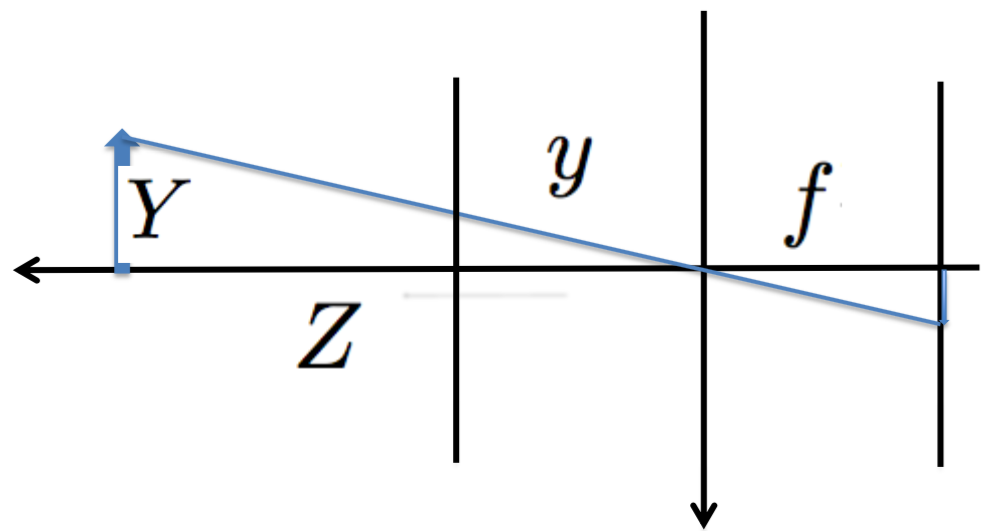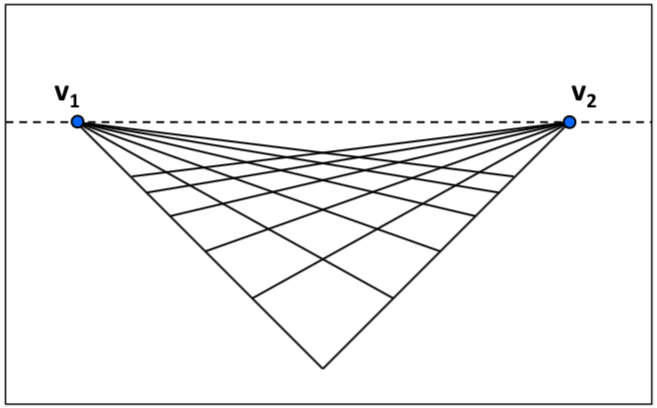## Week 1

### Pinhole Model

Assuming image plane is in front of the lens$y=f\dfrac{Y}{Z}$

### Vanishing Points• Any two parallel lines have the same vanishing point
• The ray from C through v point is parallel to the lines
• An image may have more than one vanishing point

### Vanishing Lines• Any set of parallel lines on the plane define a vanishing point
• The union of all of these vanishing points is the horizon line (also called vanishing line)
• Note that different planes define different vanishing lines

### Point and Line Duality

• Line: $$l = x \times x'$$
• Point: $$x = l \times l'$$
• Points and lines are dual in projective space: given any formula, can switch the meanings of points and lines to get another formula

### Point at Infinity

Line $$l = (a, b, c)^T$$ intersects at $$(b, -a, 0)^T$$.

### Perspective Projection

#### 1. Camera Projection Matrix

$Z_{c} \begin{bmatrix} x' \\ y' \\ 1 \end{bmatrix} = \left[ \begin{array}{ccc|c} f & 0 & 0 & 0 \\ 0 & f & 0 & 0 \\ 0 & 0 & 1 & 0 \end{array} \right] \left[ \begin{array}{c} X_c \\ Y_c \\ Z_c \\ 1 \\ \end{array} \right]$

#### 2. Intrinsic Camera Parameters

$\begin{bmatrix} x \\ y \\ z \end{bmatrix} = \left[ \begin{array}{ccc} \alpha_{x} & s & p_{x} \\ 0 & \alpha_{y} & p_{y} \\ 0 & 0 & 1 \\ \end{array} \right] \left[ \begin{array}{c} x' \\ y' \\ z' \\ \end{array} \right]$

• from optical world ( $$x', y', z'$$) to pixel world ($$x, y, z$$)
• $$\alpha_{x}, \alpha_{y}$$: pixel scaling factor
• $$p_{x}, p_{y}$$: principle point
• $$s$$: slant factor

#### 3. 3rd Person to 1st Person 3D Mapping (World to Camera)

$X_c = \left[ \begin{array}{cc} R_{3 \times 3} & t_{3 \times 1} \\ 0 & 1 \\ \end{array} \right] X$

World coordinates to camera coordinates

$x = K_{3 \times 3} [I; 0]_{3 \times 4} X_c$

Camera coordinates to pixel coordinates

$x = K [ R, t ] X$

Combine 1, 2, 3 here, we have

$Z \left[\begin{array}{c} U_{img} \\ V_{img} \\ 1 \\ \end{array}\right] = \left[\begin{array}{ccc} f_x & s & p_x \\ & f_y & p_y \\ & & 1 \\ \end{array}\right] \left[\begin{array}{cccc} r_{11} & r_{12} & r_{13} & t_1 \\ r_{21} & r_{22} & r_{23} & t_2 \\ r_{31} & r_{32} & r_{33} & t_3 \\ \end{array}\right] \left[\begin{array}{c} X \\ Y \\ Z \\ 1 \\ \end{array}\right]$

or

$\left[\begin{array}{c} x \\ 1 \\ \end{array}\right] = L \left(\begin{array}{c} K [ R \: t ] \left[\begin{array}{c} X \\ 1 \\ \end{array}\right] \end{array}\right)$

### Camera Calibration

Calibration estimates intrinsic parameters

• $$f$$ focal length
• $$(u_0, v_0)$$ image center
• $$k_1, k_2, \cdots$$ radical distortion parameters

## Week 3

### RANSAC

Problem of Minimum Square Error: outliers will affect result significantly.